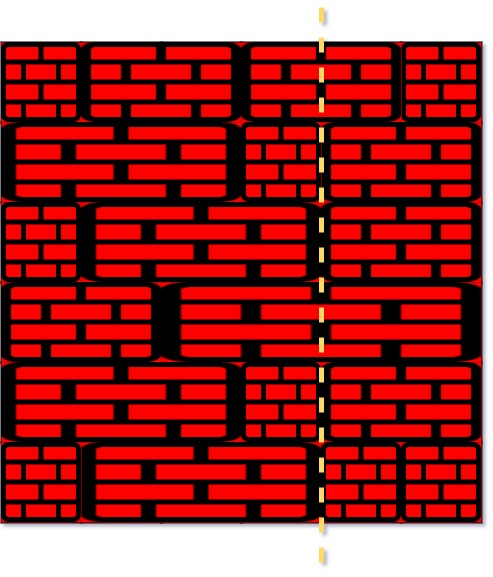# GeetCode Hub

There is a rectangular brick wall in front of you with `n` rows of bricks. The `ith` row has some number of bricks each of the same height (i.e., one unit) but they can be of different widths. The total width of each row is the same.

Draw a vertical line from the top to the bottom and cross the least bricks. If your line goes through the edge of a brick, then the brick is not considered as crossed. You cannot draw a line just along one of the two vertical edges of the wall, in which case the line will obviously cross no bricks.

Given the 2D array `wall` that contains the information about the wall, return the minimum number of crossed bricks after drawing such a vertical line.

Example 1:```Input: wall = [[1,2,2,1],[3,1,2],[1,3,2],[2,4],[3,1,2],[1,3,1,1]]
Output: 2
```

Example 2:

```Input: wall = [,,]
Output: 3
```

Constraints:

• `n == wall.length`
• `1 <= n <= 104`
• `1 <= wall[i].length <= 104`
• `1 <= sum(wall[i].length) <= 2 * 104`
• `sum(wall[i])` is the same for each row `i`.
• `1 <= wall[i][j] <= 231 - 1`

class Solution { public int leastBricks(List<List<Integer>> wall) { } }